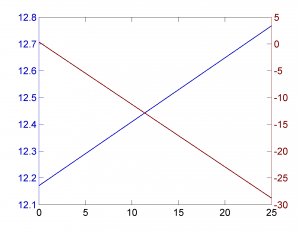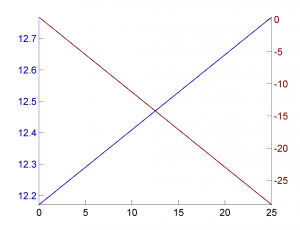Seite wählen

In matlab the normal way to create plots with two y-axis is to use the command `plotyy`.

This works quite simple, and creates equal tick locations for both data sets:

```xaxis = 0:0.1:25; y1 = linspace(12.1712,12.7679, length(xaxis)); y2 = linspace(0.3597,-28.7745, length(xaxis));   [AX,H1,H2] = plotyy(xaxis, y1, xaxis, y2);   plotstyle; % sets only colors, linestyle and fonts```However for tick that are not equal on both sides the plot looks simply wrong.

```[AX,H1,H2] = plotyy(xaxis, y1, xaxis, y2);   % axis limits - x axis (min to max) set(AX, 'XLim', [min(xaxis) max(xaxis)]); set(AX(2),'XTick',[]);   % y1 axis limits set(AX(1), 'YLim', [min(y1) max(y1)]);   % y2 axis limits set(AX(2), 'YLim', [min(y2) max(y2)]);   plotstyle;```Unfortunately the web is full of question on how to remove the ticks on the opposite side. But Matlab completely fails at this point. It is simply not supported properly.

The only solution I could find/retrieve in a forum was the following one, which is based on the idea to remove the box property which paints the ticks on the opposite side. But it also removes the surrounding box, which is unwanted.

```set(AX(1),'Box','off') % Turn off box of axis 1, which removes its right-hand ticks set(AX(2),'Box','off') % Turn off box of axis 2, which removes its left-hand ticks```Another idea I found in this thread is to work with dummy axes, but I do not see how to integrate it with my plot function (the plot is empy after I execute this code)

```% dummy axes for the box and background color ax0 = axes; set (ax0, 'Box', 'on', 'Color', 'white', 'XTick', [], 'YTick', []); % first axes for left y-axis ax1 = axes ('Position', get (ax0, 'Position')); set (ax1, 'Box', 'off', 'Color', 'none', 'YAxisLocation', 'left'); % second axes for right y-axis assuming common x-axis controlled by ax1 ax2 = axes ('Position', get (ax0, 'Position')); set (ax2, 'Box', 'off', 'Color', 'none', 'XTick', [], 'YAxisLocation', 'right');```